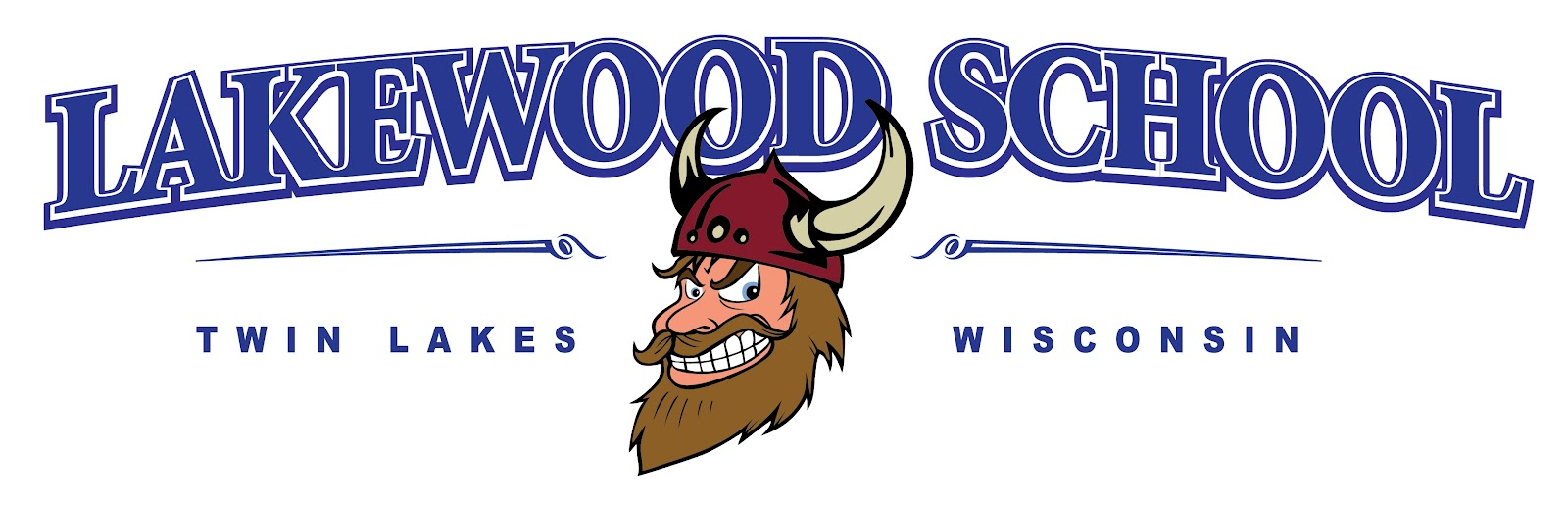• Select a School
• LanguageThird Grade Curriculum Printable ELA English, Language Arts I know the meaning of common prefixes and suffixes. I can decode words with common Latin suffixes. I can decode multisyllable words. I can read 3rd grade sight words. I can read on-level text for understanding. I can read on level text, including poetry, fluently. I can use context to help me read and self-correct if necessary. I can refer to the text when answering questions. I can determine the message of a text and use key details to explain. I can describe characters and their influence on the sequence of events. I can distinguish when I have to infer based on information in the text. I can separate my point of view from that of a narrator/character. I can compare and contrast books in the same series. I can refer to the text when asking or answering questions. I can explain how details support the main idea. I can use text features and search tools to locate information. I can explain my own point of view compared to an author's. I can use illustrations and words in text to demonstrate understanding. I can describe connections in a text. I can compare and contrast key details in two texts of the same topic. I can use an organizational structure to state my opinion and reasons using linking words. I can write about a topic, group information together with linking words and include illustrations if needed. I can write a narrative with characters using dialogue or descriptions to develop experiences and the response of the characters. MATH Operations and Algebraic Thinking, Numbers and Operations in Base Ten, Measurement and Data, Geometry Interpret products of whole numbers Interpret whole-number quotients of whole numbers Use multiplication and division within 100 to solve word problems Determine the unknown whole numbers in a multiplication or division equation relating three whole numbers Understand division as an unknown-factor problem Fluently multiply and divide within 100 Solve two-step word problems using the four operations. Represent these problems using equations with a letter standing for the unknown quantity. Assess the reasonableness of answers using mental computation and estimation strategies including rounding. Use place value understanding to round whole numbers to the nearest 10 or 100 Fluently add and subtract within 1,000 Tell and write time to the nearest minute and measure time intervals in minutes Solve word problems involving addition and subtraction of time intervals in minutes Draw a scaled picture graph and scaled bar graph to represent a data set with several categories Generate measurement data by measuring lengths using rulers marked with halves and fourths of an inch. Show the data by making a line plot, where the horizontal scale is marked off in appropriate units-whole numbers, halves, or quarters. Recognize area as an attribute of plane figures and understand concepts of area measurement Relate area to the operations of multiplication and addition Understand a fraction 1/b as the quantity formed by 1 part when a whole is partitioned into b equal parts; understand a fraction a/b as the quantity formed by a parts of size 1/b Understand a fraction as a number on the number line; represent fractions on a number line diagram Compare two fractions with the same numerator or the same denominator by reasoning about their size. Recognize that comparisons are valid only when the two fractions refer to the same whole. Record the results of comparisons with the symbols >, =, <, and justify the conclusions Understand that shapes in different categories (e.g. rhombuses, rectangles, and others) may share attributes (e.g., having four sides), and that the shared attributes can define a larger category (e.g., quadrilaterals). Recognize the rhombuses, rectangles, and squares as examples of quadrilaterals, and draw examples of quadrilaterals that do not belong to any of these subcategories Partition shapes into parts with equal areas. Express the area of each part as a unit fraction of the whole. For example, partition a shape into 4 parts with equal area, and describe the area of each part as ¼ of the area of the shape